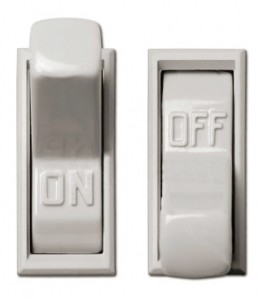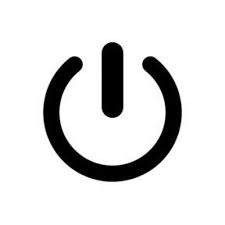# Homeschool Technology Lesson: 1 BinaryI did the first homeschool technology lesson today. I don’t know if I’ll be able to post many and I don’t expect it will be too verbose, but here goes…

## Homeschool Technology Lesson: Binary

I started by showing the boys a light switch and talking about on and off. Those are 2 ‘states’ of the light switch. It can only be one or the other. I talked a little about how the switch works on the inside: when the switch is on, the connection is made and the electricity can flow.

Then I asked about other things that can be one thing or the other: eyes open or closed, day or night, etc.

Then I asked if they could find other on/off switches in the room. They found things like a lamp, TV, etc. I focused on an on/off button they found.

I pointed out that the symbol on the on/off button has a circle and a line on it – a zero and a one. I asked which they thought the zero represented (off) and the one (on).Electronics have 2 states: on or off, zero or one – that’s digital and that’s the core of binary.

I explained how decimal numbers count from 0 to 9. Then, to go higher, they put a 1 in the next column – the tens column – and it starts over: 10, 11, etc. It’s base-10.

Other counting can be based on other numbers so a base-5 number system would go: 0, 1, 2, 3, 4 and then 10… and it would be 5 (decimal). “10” has a 1 in the “fives” column. Hold on tight!

To write ten in base-5 would be 20 (pronounced “two-zero”). It’s not twenty – it’s ten. There’s a two in the fives column.

Base-8 (octal) counting would be 0, 1, 2, 3, 4, 5, 6, 7, 10 and “10” is 8 (decimal). That’s a one in the “eights” column. Mind blown.

So binary (bi meaning 2) is base-2 so it’s 0, 1, 10 and 10 is 2 (decimal). So 11 (binary) is 3 (decimal).

Two things people tend to have a hard time with is 1) that base-10 numbering isn’t the only number system and 2) one is not the first number (Spoiler: zero is – zero is first, one is second, etc.).

My older son asked about a counting system where things double. I said “That’s binary!” We used to double things a lot and I explained it’s binary. Apparently it stuck a bit.

So in binary 1 is 1 (decimal), 10 is 2 (decimal), 100 is 4 (decimal) and so on – they double. And in binary 100 + 10 + 1 would be 4 + 2 + 1 in decimal so it would be 7. In binary, it’s 100 + 10 + 1 = 111 (7 in decimal).

But be careful not to say “ten in binary is two in decimal” – instead say “one-zero in binary is two in decimal.” or “one-one-one in binary is seven in decimal.”

“So in binary one is 1 (decimal), one-zero is 2 (decimal), one-zero-zero is 4 (decimal) and so on – they double. And in binary one-zero-zero plus one-zero plus one would be 4 + 2 + 1 in decimal so it would be 7 (decimal). In binary, it’s one-zero-zero plus one-zero plus one is one-one-one (7 in decimal).”

10 is pronounced “one-zero” (not ten) – ten in binary is 1000 (one-zero-zero-zero)

Of course another way to say it is…

“So in binary one is one, two is two…” – They’re the same values so you’d say the same thing.

It works better if you understand and can explain it. In this post I feel like I’m explaining it and explaining how I explained it and it just sounds terrible. 🙂

There was a lot of interruptions and confusion. I don’t expect them to completely get it the first time, but it’s planting seeds. Also, there was a lot of drawing on the board of the on/off button and binary stuff.

I tried to make it a combination of fun, applicable (switches around the room) and the basis of knowledge – binary is how data is stored in a computer: files, images, video, games, etc.

The intention is to build on that to explain how computer memory (RAM, hard drives, DVDs, etc.) stores things with tiny switches or other way to show on/off (binary). Those value add up to values which represent numbers, letters, etc. and everything else stored on a computer: music, video, games, etc. All based on 0’s and 1’s.